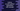# Split the root, extension of a path in Python using os.path.splitext## How to split the root and extension of a path in Python using os.path.splitext:

Python os module provides different operating system related utility functions. os.path provides different utility methods for different pathname manipulation operations.

os.path.splitext method is used to split the pathname in two parts: the root part and extension. In this post, we will learn how to use os.path.splitext method to split the root and extension of a path in Python with example.

### Definition of os.path.splitext:

os.path.splitext method is defined as like below:

``os.path.splitext(path)``

It takes one parameter here: a path name or a path like object as a tuple. It splits the path to a pair of root part and extension so that if we combine or add both, it gives the provided path.

If there is no extension in the path, it gives one empty string.

It is a really easy way to get the extension from a path in Python.

## Example of os.path.splitext:

Let’s take a look at the below example on how to use os.path.splitext with different paths:

``````import os

path_1 = '/users/code/doing/example.py'
path_2 = '/users/images/bird.png'
path_3 = '/users/programs/setup.exe'
path_4 = '/users/code/doing/'

print(os.path.splitext(path_1))
print(os.path.splitext(path_2))
print(os.path.splitext(path_3))
print(os.path.splitext(path_4))``````

If you run this program, it will print the below output:

``````('/users/code/doing/example', '.py')
('/users/images/bird', '.png')
('/users/programs/setup', '.exe')
('/users/code/doing/', '')``````

As you can see here, for path_1, path_2, path_3 and path_4, it gives the root path name and extension.

For path_4, it gives one empty string as the path is not pointing to a file with an extension.

### Reading the extension values:

Since the return type is tuple, we can read the extension value by using the index. Let’s try to print all extensions for the above program:

``````import os

path_1 = '/users/code/doing/example.py'
path_2 = '/users/images/bird.png'
path_3 = '/users/programs/setup.exe'
path_4 = '/users/code/doing/'

print(os.path.splitext(path_1))
print(os.path.splitext(path_2))
print(os.path.splitext(path_3))
print(os.path.splitext(path_4))``````

It will print:

``````.py
.png
.exe``````

As you can see here, the last one returns an empty string.

### Multiple separators:

If we have multiple periods like image.path.png, it will consider only the last period:

``````import os

path_1 = '/users/code/doing/example.second.py'

print(os.path.splitext(path_1))``````

It will print .py.

## Example with file name:

If we pass a file name to os.path.splitext, it treats that filename same as like a path and splits the contents.

``````import os

path_1 = 'image.png'

print(os.path.splitext(path_1))``````

It will split it into image and .png. If you run this program, it will print:

``.png``